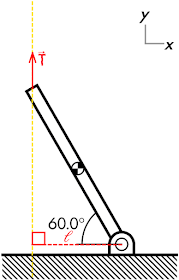## 20151121

### Physics quiz question: tension force of string on suspended beam

Physics 205A Quiz 5, fall semester 2015
Cuesta College, San Luis Obispo, CAA uniform beam (0.60 m length) is suspended by a string that pulls vertically upwards, exerting a torque of magnitude 1.1 N·m. (Calculate all torques with respect to the pivot, located at the other end.) The tension in the string is:
(A) 3.7 N.
(B) 0.92 N.
(C) 0.66 N.
(D) 1.8 N.

Correct answer (highlight to unhide): (A)The tension force T of the string acts at the top of the beam, along the string. The perpendicular lever arm ℓ for the string tension force must extend from the pivot to perpendicularly intercept the tension force line of action (which lies along the string itself), such that this will be a horizontal line of length:

ℓ = L·cos(60.0°).

The (clockwise) torque exerted by the string on the beam is then:

τ = T·ℓ,

such that the magnitude of the tension force T can be solved for:

T = τ/ℓ/ = τ/(L·cos(60.0°)),

T = (1.1 N·m)/((0.60 m)·cos(60.0°)) = 3.66666666... N,

or to two significant figures, the tension in the string is 3.7 N.

(Response (B) is τ/(2·L); response (C) is τ/2; and response (D) is τ/L.)

Sections 70854, 70855, 73320
Exam code: quiz05bL8D
(A) : 14 students
(B) : 2 students
(C) : 19 students
(D) : 30 students

Success level: 45%
Discrimination index (Aubrecht & Aubrecht, 1983): 0.47